Fermentation Tube Test Statistics for Indirect Water Sampling | OMICS International
Hydrology: Current Research

Like us on:

# Fermentation Tube Test Statistics for Indirect Water Sampling

 Marek Nawalany* and Malgorzata Loga Warsaw University of Technology, Faculty of Environmental Engineering, Nowowiejska 20, 00-653 Warsaw, Poland Corresponding Author : Marek Nawalany Warsaw University of Technology Faculty of Environmental Engineering Nowowiejska 20, 00-653 Warsaw, Poland Tel: 22-234-74-25 E-mail: [email protected] Received January 09, 2014; Accepted February 18, 2014; Published February 21, 2014 Citation: Nawalany M, Loga M (2014) Fermentation Tube Test Statistics for Indirect Water Sampling. Hydrol Current Res 5:165. doi: 10.4172/2157-7587.1000165 Copyright: © 2014 Nawalany M, et al. This is an open-access article distributed under the terms of the Creative Commons Attribution License, which permits unrestricted use, distribution, and reproduction in any medium, provided the original author and source are credited. Related article at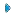PubmedScholar Google

Visit for more related articles at Hydrology: Current Research

#### Abstract

By aiming at correction of the existing standards of the Fermentation Tube Test (FTT) this article critically reviews one of the oldest statistical methodologies used in sanitary engineering clearly relevant to health science. The common practice of water works is to perform the FTT on water samples for detecting fecal bacteria contamination in raw water prior to technological processing. Analysis of the Fermentation Tube Test (FTT) statistics presented in the article is to support a hypothesis that “standard FTT procedures may not be compatible with the statistical tables of FTT in the Standard Methods (1998, 2005)”. The inconsistency can be seen from the observation that the standard FTT procedures require subsequent dilution of water samples, which implies the indirect sampling. At the same time, the Standard Methods (1998, 2005) statistical tables used for FTT interpretations result from the assumption of the direct water sampling. In the article a statistical context of the Most Probable Number of bacteria, MPN, for actual, i.e. indirect, procedures of water sampling is described. Theoretical background of the inconsistency is explained and the remedy proposed by means of a new formula for calculating Most Probable Number of Bacteria consistent with actual indirect sampling procedures. The inconsistency is illustrated with simple but realistic example. As the ultimate result of the research it is proposed to modify the existing MPN tables and thus eliminate the inconsistency between the standard FTT procedures and the FTT tables published in the Standard Methods (1998, 2005) and ISO (1988) standards.

 Keywords Bacteria content in water; Fermentation tube test; Most probable number of bacteria; Bayesian interpretation; Direct sampling; Indirect sampling; Inconsistency in standard methodology; Monte Carlo Introduction To protect population against waterborne diseases many water treatment plants use the Fermentation Tube Test (FTT) to assess bacterial pollution of raw water. This simple monitoring technique is based on repetitive sampling of water with a set of standard tubes, followed by adding lactose to the water samples and counting samples from which fermentation gas is released. Gas that results from lactose consumption by bacteria can be easily detectable, which makes the FTT an attractive measuring technique. Number of fermenting tubes is a basis for calculating the Most Probable Number of bacteria (MPN), classical measure of bacteriological content in water. It seems that little has changed from the time when well known article on MPN was published by Cochran . Up to now many researchers apply the Most Probable Number approach when referring to water sanitary standards [2,3]. The concept has been adopted also by food industry . Recently the Most Probable Number Calculator has been proposed by US EPA  to simplify calculations of MPN. It enables usage of different experimental tube volumes, number of tubes and performs calculations of confidence intervals for different confidence levels. Apart from MPN value, the program can also compute: the Spearman-Kraber estimate, the bias correction and the confidence limits described by Cornish et al. , and Loyer et al, . Although of practical importance all these refinements were based on FTT procedures and interpretations described in consecutive editions of Standard Methods, (SM). These statistical interpretations of MPN has been challenged by new, Bayesian, approach to the Fermentation Tube Test (FTT), introduced by Nawalany  and Nawalany et al. . The approach was also followed by Nawalany et al.  in the article concerning the FTT statistics for direct and independent water sampling. Also there a new equation for the most probable concentration of bacteria has been derived. This enabled to estimate a magnitude of uncertainty that results from calculating MPN using so called Thomas formula. Although very inaccurate in some instances, the formula is still recommended by the Standard Methods [11,12]. Present analysis of the FTT statistics is to support a hypothesis that “standard FTT procedures may not be consistent with the statistical tables of FTT in SM”. The tables have been derived decades ago under assumption of direct water sampling whereas present FTT standards [11,12] require subsequent dilution of water samples which implies indirect sampling. Concern about the associated health risk ascertains importance of correcting formulae for assessing bacteriological status of natural waters. In this article statistical context of MPN for actual (i.e. indirect) water sampling is presented. After quantifying differences in probabilities of getting particular FTT outcomes for direct and indirect sampling it is proposed to modify the MPN statistical tables of SM and ISO. The ultimate goal of this article is to remove present inconsistency between the standard FTT procedures and the FTT tables published in the Standard Methods and ISO standards. Below the FTT procedures and the selected formulae from previous papers [8-10] are shortly recalled to make the article self-contained. Large containers are normally used to take water samples directly from natural water sources (lakes, rivers, etc.) at some fixed locations. Together with water sample of volume Vo some finite number of bacteria - No, may fall into the container as a result of random draw. Then, water in the container is sampled with a small tube of volume Vt. The container is thus becoming a water source for the subseq sampling operations. Some bacteria from this water source may fall into the volume Vt in the moment of sampling. If volume Vo of water in the source is sufficiently large and well mixed, probability that some bacterium from the water source falls into the volume Vt is equal to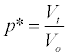(1) By adding lactose to the water sample of volume Vt one initiates the FTT test. Gas produced by bacteria consuming lactose is the indicator of possible bacteriological pollution of water. It is assumed hereafter that the test is ideal, i.e. after adding lactose the outcome of FFT is “positive” (fermentation gas is released) if and only if there is at least one bacterium in the water sample and is “negative” (fermentation gas is not released) if and only if water is sterile (i.e. there is no even one bacterium in the sample). Formula (1) can be generalized for the situations when not just one bacterium but k bacteria fall into the sampling volume. As bacteria are supposed to move in water independently of each other, a probability that exactly k bacteria fall into the sampling volume Vt at the moment of sampling is represented by binomial probability distribution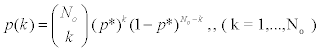(2) When average concentration of bacteria in the water source is equal to n, No in formula (2) can be replaced by No = nVo . After substituting formula (1) for p* into (2) one obtains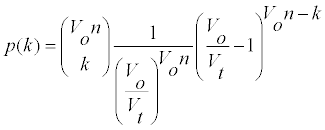(3) Consequently, respective probabilities of getting “positive” or „negative” outcome from FTT are equal to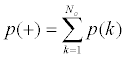(4a)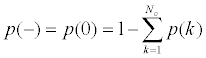(4b) Formulae (3) and (4) are used in next paragraphs to analyze statistical dependencies of the FTT outcomes that arise when water is sampled with the standard procedures, i.e. by indirect sampling. Straightforward consequence of formula (3) is that probability of falling exactly k bacteria into the sampling volume Vt converges to the Poisson formula when ratio Vo/Vt tends to infinity, i.e.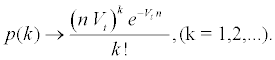(5) In practical applications Vo is always considerably larger than Vt and this justifies of using the Poisson formula (5) for p(k) in standard FTT tables. Repeated Indirect Sampling Actual realization of the FTT follows the standard mode of water sampling described in the Standard Methods [11,12]. This mode definitely must be classified as indirect. Below probabilities of “positive” or “negative” outcome of the FTT for repeated indirect sampling are derived in two steps. In the first step probability of getting specified number of bacteria into a tube is derived whereas in the second step formulae for “positive” and “negative” outcomes of the FTT are followed by the ultimate equation from which the MPN can be calculated. Probability of getting specified number of bacteria According to the standard procedure only the first tube of volume Vt samples water directly from the water source (water body). Mixing the sampled water with somewhat larger volume of sterile water makes the resultant diluted water ready for subsequent sampling. The mix is normally kept within some container of volume Vp from which the next tube samples water. Water of the secondary sampling is also diluted and contained within yet another container. The procedure – of taking sample of (already diluted) water with a tube, diluting it and confining in the next container – is repeated along the chain of jo containers. Number of bacteria in a given (secondary) container depends on bacteriological status of water samples drawn from the previous containers. Water laboratories of water companies routinely apply the FTT test using sequential (or cascade) dilution of water samples to examine bacteriological status of natural waters. This type of sampling being the indirect sampling of the water source implies necessity of introducing new formulae for probability of getting positive FTT outcome. Clearly, the existing formulae for direct sampling  must be essentially modified to account for indirect sampling and water dilution. Formulae in the next paragraphs refer to Figure 1 presenting a scheme of the FTT procedure based on dilution of consecutive samples of water. Indirect water sampling is routinely repeated number of times, say, r-times. Each time, lactose is added to samples of diluted water in the containers. When fermentation gas is released from the j-th sample, the FTT is said to give a “positive” outcome. Number of positive outcomes observed in the j-th container when the test is repeated r-times is denoted as mj. For any j-th sample, (j = 1,..,jo), mj can be 0 or 1 or 2,..,or r. A jo-tuple (m1, m2,...,mjo) represents an integrated outcome of FTT when the test is repeated r-times on jo water samples. One-time dependent sampling can be described as follows. Let Vt denote a volume of each sampling tube. The first tube samples from large volume of the water source - Vo. In this volume of water concentration of bacteria is assumed to be equal to n. The water sample of volume Vt drawn from the water source is added to, mixed and diluted with sterile water of volume [10κ-1]Vt confined in some container. This way volume of diluted water sample in this container becomes equal to 10κVt. Parameter κ indicates the order of dilution. According to standard procedures, κ = 1, i.e. water samples are diluted 10-times. Next step is of taking a sample of volume Vt from the container confining volume Vp = 10Vt of diluted water. Sampled water is then diluted with sterile water of volume 9Vt and confined in yet another container resulting in identical volume of diluted water, i.e. Vp = 10Vt. Water in each container is well mixed before next sample is drawn. A procedure of a) sampling water from the previous container of volume Vp = 10Vt using a tube of volume Vt b) diluting the sampled water with sterile water of volume 9Vt and c) confining it in next container of volume 10Vt is repeated number of times. For instance, number of containers of volume Vp is equal to jo = 5 and, hence, steps a) – c) are repeated jo–1 = 4 times. Only the first draw (from the water source) satisfies condition of sampling from a large water volume. The following draws, which are taken from volumes Vp = 10Vt, need to be considered as sampling from the finite (and rather small) volumes of water. This observation has its consequences when deriving formulae for probability of getting a positive outcome of FTT (i.e. getting at least one bacterium in the sampling tube) when sampling from the i-th container. To evaluate probability of “success” the following notations are introduced: Ki – number of bacteria present in the i-th container, (i = 1,.., jo), after sampling with a tube of volume Vt from the previous (i-1)-th container, but before the i-th container is sampled with the next (i+1)- th tube. The water source is considered to be container number “0”. ki - number of bacteria which are left in the i-th container, (i = 1,.., jo), after the i-th container is sampled with the next (i+1)-th tube. Clearly, for i = jo the corresponding kjo = Kjo. ni – concentration of bacteria within the i-th container before the container is sampled with the next tube of volume Vt for dilution in the next, (i+1)-th container. This concentration is equal to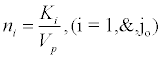(6) where Vp = 10Vt – volume of water in the i-th container (before it is sampled by the next tube). As ratio Vp/Vt is rather small, formulae (3) and (4), appropriate for sampling from small volumes, must be applied for calculating probability of a “success” – pi(+), (i = 1,.., jo). Sampling from the water source to the first container followed by consecutive dilutions in jo containers results in a jo-tuple representing number of bacteria ultimately remaining in the containers – (k1, k2, .., kjo), where ki can be any non-negative integer - 0,1,2,... It must be noted that in order to create particular tuple (k1, k2, .., kjo), sampling from the water source to the first container should result in drawing exactly (k1+ k2+ ..+ kjo) bacteria, sampling from the first container to the second container should result in drawing exactly (k2+ ..+ kjo) bacteria, sampling from the second container to the third container should result in drawing exactly (k3+ ..+ kjo) bacteria, and so on.. The corresponding partial sums of bacteria denoted as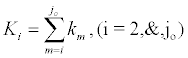(7) represent number of bacteria that are passed to the i-th container as a result of sampling water with the tube of volume Vt from the previous (i-1)-th container. The pool of bacteria Ki, (i = 1,..,jo) is then redistributed in the containers i,i+1,.., jo as a result of subsequent sampling and dilution. Conditional probability p(k1, k2, .., kjo|n) of getting a particular tuple (k1, k2, .., kjo) when concentration of bacteria in the water source is equal to n can be calculated as a product of conditional probabilities: of taking K1 bacteria from the water body provided concentration of bacteria in the original water body is equal to n, of taking K2 bacteria from the first container provided concentration of bacteria in the container is equal to n1, of taking K3 bacteria from the second container provided concentration of bacteria in the container is equal to n2, and so on... Hence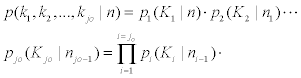(8) where no ≡ n. Formula (8) takes into account a dependence of getting particular number of bacteria within the chain of containers as the result of sequential sampling and dilution. It can be observed that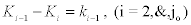(9a) b) Kio = kjo . Sampling water with a tube of volume Vt (e.g. Vt = 1ml) from the water source allows to assume that Vo / Vt → ∞. Consequently, probability that exactly K1 bacteria falls into the first container can be expressed by the Poisson probability (5):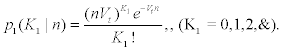(10) When there are exactly K1 bacteria in the first container (within water volumeVp =10 ⋅Vt ) then the corresponding concentration of bacteria in this container is equal to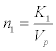(11) What is probability p2(K2 | n1) of getting exactly K2 bacteria within volume Vt of water taken from the first container for dilution in the second one? To calculate probability p2(K2 | n1) one must use formula (3) as the only formula suitable for finite (and rather small) ratio Vp / Vt. In this phase of procedure volume Vp =10Vt plays a role of Vo whereas n1 corresponds to bacteria concentration n in formula (3). In general case, the probability of passing exactly Ki bacteria into the i-th container from the container (i-1)-th is equal to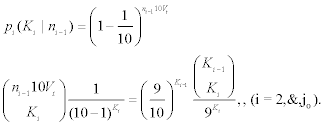(12) When there are no bacteria in the (i-1)-th container, (i.e. when ni-1 = 0 or equivalently, when Ki-1 = 0), probability of passing nonzero number of bacteria to the i-th container is equal to zero whereas probability of passing no bacteria is then equal to 1, i.e.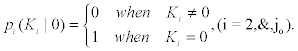(13a) Generally, one cannot pass more bacteria to the i-th container than the number of bacteria, which are actually confined in the (i-1)-th container before it is sampled, i.e.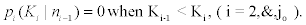(13b) Constraints (13a) and (13b) are satisfied automatically as the Newton symbol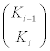in formula (12) is by definition equal to zero when Ki-1 < Ki and equal to 1 when Ki-1 = Ki. Formulae for “positive” and “negative” outcomes of the FTT In order to evaluate probability of getting particular result of the repetitive FTT - jo-tuple (m1 ,.., mjo), one must first calculate probability of getting a “success” on any j-th position of the tuple when FTT is realized one time on water samples in all - jo, containers. This probability is equal to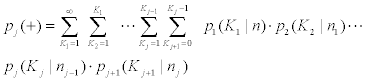for j = 1,...,jo-1                         (14a) and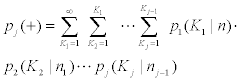for j = jo                                   (14b) where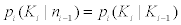are given by formulae (11) –(13). Interpretation of formulae (14) is quite straightforward. They mean that in order to get a “success” on the j-th position of the jo-tuple two independent events must take place: 1. at least one bacterium is passed from the proceeding containers to the j-th container 2. sampling for the next, (j+1)-th container does not result in taking all bacteria from the j-th container. Substitution of formula (12) into formulae (14) shows that a dependence of pj(+) on n is only due to dependence of the first draw FTT outcome on n: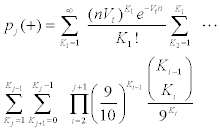for j = 1,...,jo-1                                                               (15a)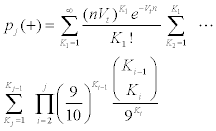for j = jo.                                                                        (15b) Probability of getting a “negative” outcome on the j-th position of the jo-tuple is equal to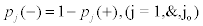Furthermore, a derivative of probability (15) is given by following formula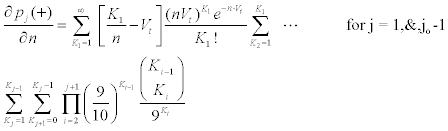(17a)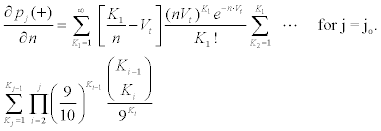(17b) When the indirect sampling procedure is repeated r-times a probability of getting mj ”successes” at the j-th position of the jo-tuple is given by the following formula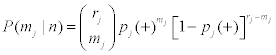(18a) whereas probability of getting particular tuple (m1, m2, .., mjo) is given by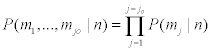(18b) For FTT tests with indirect sampling and sequential dilution repeated r-times most probable concentration of bacteria n* must be calculated using formula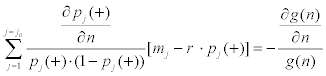(19) with pj(+) calculated from formulae (15) and derivative of pj(+) from formulae (17); g(n) is a-priori probability of concentration n of bacteria in the water source. It has been experienced that although the infinite series in formulae (15) and (17) are always approximated with finite sums, it takes prohibitively long time to compute the formulae when summing up too many terms in the first sum (the computational effort increases exponentially with the number of terms in approximating sum). This problem has been dealt with and overcome using Monte Carlo approach – see the following paragraph. It can be proven from formula (15) that in case of using sampling tubes having equal volumes Vt, probability of the successful outcome of the FTT test in the j-th water sample is smaller in case of indirect sampling than the corresponding probability of success for the direct sampling, i.e.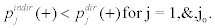(20) Correcting Standard MPN Tables Procedures for the FTT described in international standards [11,12] are based essentially on multiple indirect water sampling. But statistical interpretation of the test and formulae used to calculate Most Probable Number of bacteria (MPN) are based on the assumption of direct sampling! In this paragraph the discrepancy between commonly accepted statistical interpretation of the FTT test (based on independent direct sampling) and its actual execution (based on indirect sampling) is demonstrated. It is shown that standard statistical tables underestimate the measure of bacteria content in water - MPN. To support this statement a simple but realistic example is presented Let analysed water is sampled with 5 tubes of volume Vt =10ml and diluted 4 times. Consecutive dilutions are in proportion 1:10, i.e. water of each tube of Vt =10ml is mixed with 9Vt volumes of sterile water. Also, two repetitions of the procedure are made, i.e. r = 2. Following notation introduced by Nawalany et al  this FTT test is to be denoted FTT (2,2,2,2,2). Accordingly, for calculating MPN, 5-tuples (m1,.., m5) of FTT outcomes are used. In Table 1, values of MPN calculated using different interpretations of FTT are presented in columns for seventeen 5-tuples staring from the 5-tuple (2,2,0,0,0). Some initial 5-tuples have been omitted as these initial 5-tuples are considered very unlikely to occur. Table 1 contains the following values of MPN: MPN5 direct - according to direct sampling formulae for 5-tuples , MPN5 indirect - according to standard indirect sampling and finally, MPN5 indirect- MC according to M-C simulation of indirect sampling. The latter estimate of the MPN has been introduced after unsuccessful computations of the MPN from equation (19) and formulae (15)-(17). The infinite series in formulae (15) and (17) are converging very slowly. They were actually applied only for first five MPN5 indirect values. For the next tuples, computational effort started to grow exponentially thus stopping computations completely. As a remedy, Monte-Carlo model have been developed which allowed for simulating a random events having probability of “success” equal to the theoretical formulae (15), i. e. describing probability of FTT “success” on all positions of the 5-tuple for the case of indirect sampling. Also corresponding values of MPN (MPNindirect - M-C) for indirect sampling have been effectively calculated with this technique. Thanks to its high efficiency, the Monte- Carlo model offered values of MPN missing from column (2) of Table 1. Relative error δ of calculating MPN from formulae assuming direct sampling (MPN5 direct - column 1) instead of using MPN5 indirect (column 3) ranges from 0,1 to 15,6 %. Conclusions • The most striking is an assumption of direct independent water sampling made in the Standard Methods and likewise in ISO. This assumption is not coherent with actual procedures of performing the FTT tests. Standard FTT procedures assume sequential dilution of indirectly sampled water samples. Hence, when calculating the most probable concentration of bacteria in water n* (and consequently MPN), formulae (15) – (17) should be substituted to equation (19) instead of formulae for direct independent sampling. Values of MPN corresponding to indirect sampling are contained in columns (2) – MPN5 indirect and (3) - MPN5 indirect-M-C of Table 1. • Values of MPN5 direct calculated according to the Standard Methods underestimate actual values of MPN. Exact values of MPN are those calculated for indirect sampling – columns (2) and (3) in Table 1. Comparison of values in column (3) with values of MPN5 direct in column (1) indicates that largest relative error of MPN5 direct is of order 15% - see column (4) in Table 1. Therefore, values of MPN5 indirect- M-C should ultimately substitute values of MPN published in the Standard Methods for last 80 years. Acknowledgements This work was supported by statutory grant of Warsaw University of Technology. References Cochran WG (1950) Estimation of bacterial densities by means of the “most probable number”. Biometrics 6: 105-116.  Yadav N, Singh B, Khurana SC, Kumar M, and Yadav R (2012) Seasonal Variation of Coliforms and Nitrate in Ground Water Quality in Kanpur Metro, Int J Res Chem Environ 2: 207-209. Rahman S, Parvez AK, Islam R (2013) Identification and quantification of Escherichia coli from drinking water in Bangladesh.  Int J of Microbiology and Immunology Research 1: 047-051. BAM (2010) Bacteriological Analytical Manual, Appendix 2, Most Probable Number from Serial Dilutions. EPA, Most Probable Number (MPN) (2012) User and System Installation and Administration Manual. Cornish EA, Fisher RA (1937) Moments and cummulants in the specification or distributions. Rev Int Statist Ins 5: 307-320. Loyer MW, Hamilton MA (1984) Interval Estimation of the Density of Organisms Using a Serial-Dilution Experiment. Biometrics 40: 907-916. Nawalany M (2000) Bayesian Interpretation of the Fermentation Tube Test, VUB Publications, Series - Hydrologie 37. Nawalany M, Loga M (2004) Most Probable Number of Bacteria in water revisited (the Bayesian approach), Proceedings of the ISSHA4 Stochastic Hydraulics Conference, Nijmegen. Nawalany M, Loga M (2010) Fermentation Tube Test Statistics for Independent Sampling and Comments on the Thomas Formula. Water & Health 1-8. Standard Methods for the Examination of Water and Wastewater United Book Press, Inc, Baltimore. Water quality - General guide to the enumeration of micro-organisms by culture. International Organization for Standards ISO 8199: 1988.

#### Tables and Figures at a glanceTable 1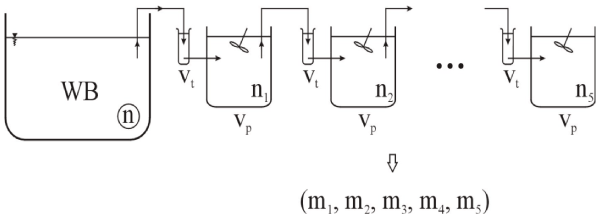Figure 1
Select your language of interest to view the total content in your interested language

### Article Usage

• Total views: 12666
• [From(publication date):
February-2014 - Jan 28, 2020]
• Breakdown by view type
• HTML page views : 8856Can't read the image? click here to refresh